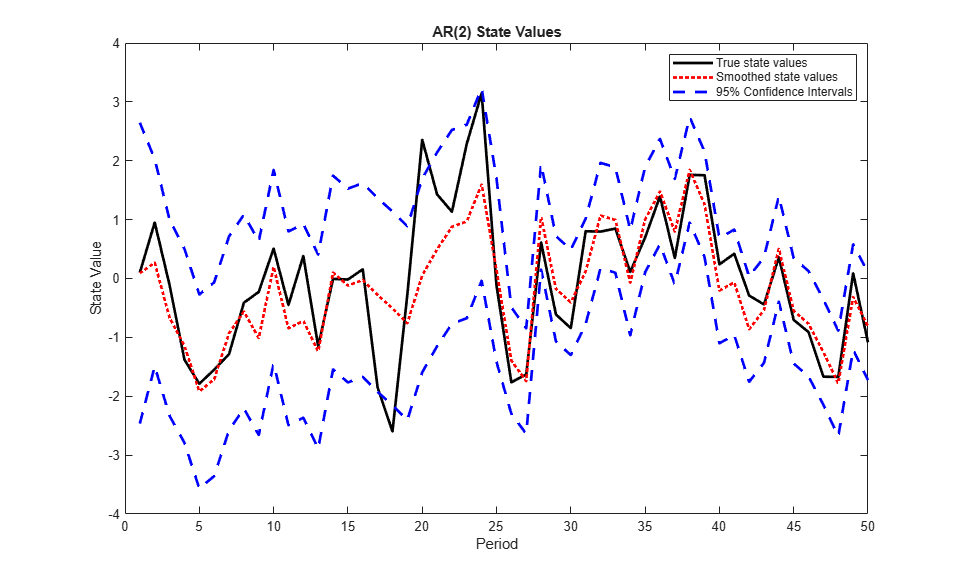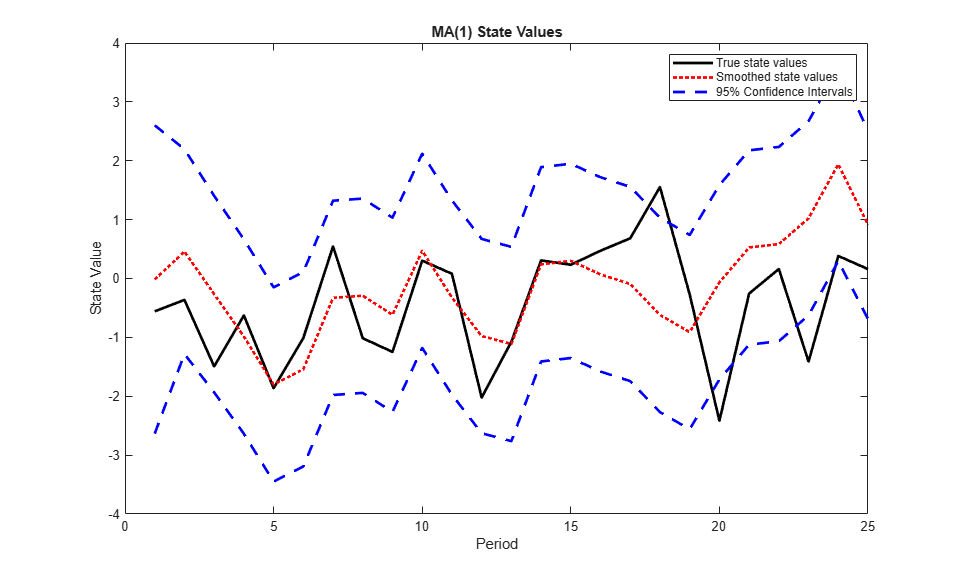Documentation

This is machine translation

Mouseover text to see original. Click the button below to return to the English version of the page.

Smooth Time-Varying State-Space Model

This example shows how to generate data from a known model, fit a state-space model to the data, and then smooth the states.

Suppose that a latent process comprises an AR(2) and an MA(1) model. There are 50 periods, and the MA(1) process drops out of the model for the final 25 periods. Subsequently, the state equation for the first 25 periods is

``

and for the last 25 periods, it is

``

whereandare Gaussian with mean 0 and standard deviation 1.

Assuming that the series starts at 1.5 and 1, respectively, generate a random series of 50 observations fromand.

```T = 50; ARMdl = arima('AR',{0.7,-0.2},'Constant',0,'Variance',1); MAMdl = arima('MA',0.6,'Constant',0,'Variance',1); x0 = [1.5 1; 1.5 1]; rng(1); x = [simulate(ARMdl,T,'Y0',x0(:,1)),... [simulate(MAMdl,T/2,'Y0',x0(:,2));nan(T/2,1)]]; ```

The last 25 values for the simulated MA(1) data are `NaN` values.

Suppose further that the latent processes are measured using

``

for the first 25 periods, and

``

for the last 25 periods, whereis Gaussian with mean 0 and standard deviation 1.

Use the random latent state process (`x`) and the observation equation to generate observations.

```y = 2*nansum(x')'+randn(T,1); ```

Together, the latent process and observation equations compose a state-space model. Supposing that the coefficients are unknown parameters, the state-space model is

``

for the first 25 periods,

``

for period 26, and

``

for the last 24 periods.

Write a function that specifies how the parameters in `params` map to the state-space model matrices, the initial state values, and the type of state.

``` % Copyright 2015 The MathWorks, Inc. function [A,B,C,D,Mean0,Cov0,StateType] = AR2MAParamMap(params,T) %AR2MAParamMap Time-variant state-space model parameter mapping function % % This function maps the vector params to the state-space matrices (A, B, % C, and D), the initial state value and the initial state variance (Mean0 % and Cov0), and the type of state (StateType). From periods 1 to T/2, the % state model is an AR(2) and an MA(1) model, and the observation model is % the sum of the two states. From periods T/2 + 1 to T, the state model is % just the AR(2) model. A1 = {[params(1) params(2) 0 0; 1 0 0 0; 0 0 0 params(3); 0 0 0 0]}; B1 = {[1 0; 0 0; 0 1; 0 1]}; C1 = {params(4)*[1 0 1 0]}; Mean0 = ones(4,1); Cov0 = 10*eye(4); StateType = [0 0 0 0]; A2 = {[params(1) params(2) 0 0; 1 0 0 0]}; B2 = {[1; 0]}; A3 = {[params(1) params(2); 1 0]}; B3 = {[1; 0]}; C3 = {params(5)*[1 0]}; A = [repmat(A1,T/2,1);A2;repmat(A3,(T-2)/2,1)]; B = [repmat(B1,T/2,1);B2;repmat(B3,(T-2)/2,1)]; C = [repmat(C1,T/2,1);repmat(C3,T/2,1)]; D = 1; end ```

Save this code as a file named `AR2MAParamMap` on your MATLAB® path.

Create the state-space model by passing the function `AR2MAParamMap` as a function handle to `ssm`.

```Mdl = ssm(@(params)AR2MAParamMap(params,T)); ```

`ssm` implicitly creates the state-space model. Usually, you cannot verify an implicitly defined state-space model.

Pass the observed responses (`y`) to `estimate` to estimate the parameters. Specify an arbitrary set of positive initial values for the unknown parameters.

```params0 = 0.1*ones(5,1); EstMdl = estimate(Mdl,y,params0); ```
```Method: Maximum likelihood (fminunc) Sample size: 50 Logarithmic likelihood: -114.957 Akaike info criterion: 239.913 Bayesian info criterion: 249.473 | Coeff Std Err t Stat Prob --------------------------------------------------- c(1) | 0.47870 0.26634 1.79733 0.07229 c(2) | 0.00809 0.27179 0.02976 0.97626 c(3) | 0.55735 0.80958 0.68844 0.49118 c(4) | 1.62679 0.41622 3.90848 0.00009 c(5) | 1.90021 0.49563 3.83391 0.00013 | | Final State Std Dev t Stat Prob x(1) | -0.81229 0.46815 -1.73511 0.08272 x(2) | -0.31449 0.45918 -0.68490 0.49341 ```

`EstMdl` is an `ssm` model containing the estimated coefficients. Likelihood surfaces of state-space models might contain local maxima. Therefore, it is good practice to try several initial parameter values, or consider using `refine`.

Smooth the states and estimate the variance-covariance matrices of the smoothed states by passing `EstMdl` and the observed responses to `smooth`.

```[~,~,Output]= smooth(EstMdl,y); ```

`Output` is a `T`-by-1 structure array containing the smoothed states and their variance-covariance matrices, among other things.

Extract the smoothed states and their variances from the cell arrays. Recall that the two, different states are in positions 1 and 3. The states in positions 2 and 4 help specify the processes of interest.

```stateIndx = [1 3]; % State Indices of interest SmoothedStates = NaN(T,numel(stateIndx)); SmoothedStatesCov = NaN(T,numel(stateIndx)); for t = 1:T maxInd1 = size(Output(t).SmoothedStates,1); maxInd2 = size(Output(t).SmoothedStatesCov,1); mask1 = stateIndx <= maxInd1; mask2 = stateIndx <= maxInd2; SmoothedStates(t,mask1) = ... Output(t).SmoothedStates(stateIndx(mask1),1); SmoothedStatesCov(t,mask2) = ... diag(Output(t).SmoothedStatesCov(stateIndx(mask2),... stateIndx(mask2))); end ```

Plot the true state values, the smoothed state values, and their individual 95% Wald-type confidence intervals for each model.

```AR2SSCIlb = SmoothedStates(:,1) - 1.95*sqrt(SmoothedStatesCov(:,1)); AR2SSCIub = SmoothedStates(:,1) + 1.95*sqrt(SmoothedStatesCov(:,1)); AR2SSIntervals = [AR2SSCIlb AR2SSCIub]; MA1SSCIlb = SmoothedStates(:,2) - 1.95*sqrt(SmoothedStatesCov(:,2)); MA1SSCIub = SmoothedStates(:,2) + 1.95*sqrt(SmoothedStatesCov(:,2)); MA1SSIntervals = [MA1SSCIlb MA1SSCIub]; figure plot(1:T,x(:,1),'-k',1:T,SmoothedStates(:,1),':r',... 1:T,AR2SSIntervals,'--b','LineWidth',2); title('AR(2) State Values') xlabel('Period') ylabel('State Value') legend({'True state values','Smoothed state values',... '95% Confidence Intervals'}); figure plot(1:T,x(:,2),'-k',1:T,SmoothedStates(:,2),':r',... 1:T,MA1SSIntervals,'--b','LineWidth',2); title('MA(1) State Values') xlabel('Period') ylabel('State Value') legend({'True state values','Smoothed state values',... '95% Confidence Intervals'}); ```